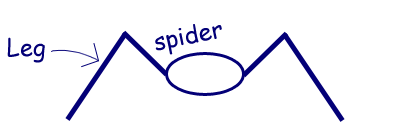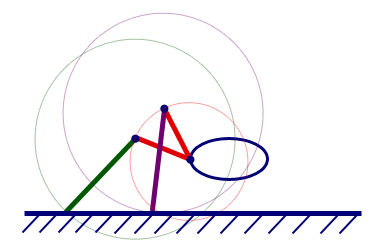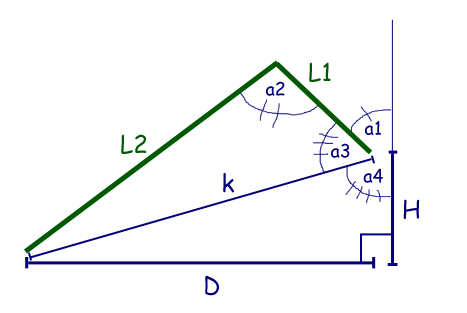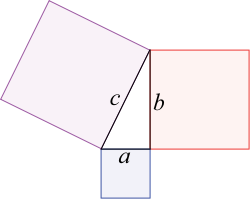## Saturday, August 22, 2009

### Spiderwheels: algebra, geometry, and trigonometry...

I've been wanting to build a spider robot for a log time. I remember looking around the internet years ago to see if other people have done this. One project really stands out in my mind.

The fluid movements of Zenta's six legged robot has inspired me to really put some thought into the mathematics behind the movement of a spider before I get too far with design and coding. After a few days of diagrams, equations, and some proofs of concept I have come up with some trigonometric functions that describe a subset of the movement of a spider. Now, let's dive in.

Consider the following:Yes. That is correct. Spiders have legs. Now, consider the following:A picture says a thousand words. What I am trying to convey with this one is that the joints that make up the leg of a spider have ranges of motion. At one given point in time the position of this leg can be described by two angles, i.e. the hip and knee, angles. These angles are key because they are "servo speak".

Now, wouldn't be good fun if we could describe the position of the leg in terms D and H in the following diagram?Considering I will be building these legs I will know L1 and L2. So, given L1, L2, D, and H, how do we find a1 and a2.

The first step is to find k. According to Pythagoras, a2 + b2 = c2.So...

k2 = D2 + H2
k = sqrt(D2 + H2).

Once we have k we can use the Law Of Cosines to find a2.

k2 = L12 + L22 - (2 * L1 * L2 * cos(a2))
cos(a2) = (L12 + L22 - D2 - H2) / (2 * L1 * L2)
a2 = cos-1( (L12 + L22 - D2 - H2) / (2 * L1 * L2) ).

Similarly, we can find a4.

a4 = cos-1( (L12 - L22 + D2 + H2) / (2 * L1 * sqrt(D2 + H2)) ).

We also know that

tan(a3) = D / H
a3 = tan-1(D / H).

Finally, we can solve for a1.

a1 = 180� - a3 - a4
a1 = 180� - tan-1(D / H) - cos-1( (L12 - L22 + D2 + H2) / (2 * L1 * sqrt(D2 + H2)) ).

In my next blog entry I will show a video of some proof of concept work, as well as share the source code that makes it all happen.